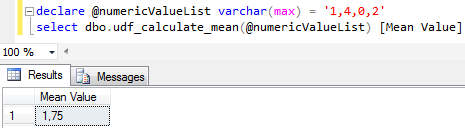﻿ Calculate Mean Value in SQL ServerSQL Server and T-SQL Development Tutorials Development resources, articles, tutorials, code samples, tools and downloads for ASP.Net, SQL Server, Reporting Services, T-SQL, Windows, AWS, SAP HANA and ABAP

# Calculate Mean Value in SQL Server

SQL programmers can calculate mean value of a numeric array in SQL Server by using SQL Server AVG() aggregate function. Transact-SQL developers or database administrators can use mean value calculation method on any numeric table column in a SQL Server database as shown in this SQL tutorial.

First of all let SQL database administrators create database table to store sample numeric values where programmers can calculate the mean value of the numeric values.

Let's now generate sample data and populate sql table.
SQL developers and database administrators can to calculate the mean value of the values stored in this table column. Here is a SQL script which will create 5 random numbers using SQL Rand() function and insert into database table.

Here is the source codes of the SQL Select script which can be used to calculate the mean value of a numeric value list given on SQL Server.
What is important about the below SQL mean value calculation using AVG() SQL aggregate function is multiplying the values with 1.0 beforehand for decimal value result. As seen below the following two average calculations return integer values losing the decimal points return false values.

Here is a screenshot of the above mean calculation or average calculation in SQL Server.Calculate mean value of an array in SQL Server

It is also possible to create a user defined SQL function which takes the numeric values as a string input parameter formed of concatenated numeric values and returns the mean value as follows.

Since SQL aggregate function does not accept multiple values I had to create this SQL mean value calculation function.

In the Mean calculation function, a second user defined SQL function dbo.split() is used to split concatenated numeric values which are passed as input to the SQL mean function. SQL Server developers and administrators can check SQL tutorial SQL Split function for the source codes of the helper function.

And this sample SQL function can be used to calculate mean value of a numeric list or average value of items as given in the following example on SQL ServerMean value (average) calculation function for SQL Server developers

SQL Server